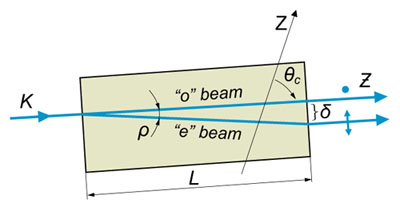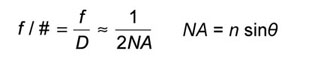EKSMA Optics uses cookies to give you the best shopping experience. If you continue to use our services, we will assume that you agree to the use of such cookies.

0
There are no items in your cart.
Add items to your cart and request an official quote or order online
Subtotal
0 \$
0
Products categories
There are no items in your cart.
Add items to your cart and request an official quote or order online
Subtotal
0 \$

EKSMA Optics uses cookies to give you the best shopping experience. If you continue to use our services, we will assume that you agree to the use of such cookies.

# Formulas & Tools

Refractive Index Dispersion Equations & Calculator
Useful Formulas & Constants
Formula
ne2 = 2.3849 – 1.259*10-22 + 1.079*10-22 + 1.6518*10-44 - 1.94741*10-66 + 9.36476*10-88
no2 = 2.35728 – 1.17*10-22 + 1.054*10-22 + 1.34143*10-44 – 4.45368*10-76 + 5.92362*10-88
n2 = 1 + 0.6961663*λ2/(λ2 – 0.00467914826) + 0.4079426*λ2/(λ2 – 0.0135120631) +0.8974794*λ2/(λ2 – 97.9340025)

n2 = 1 + 1.03961212*λ2/(λ2 – 0.00600069867) + 0.231792344*λ2/(λ2 – 0.0200179144) +1.01046945*λ2/(λ2 – 103.560653)

n2 = 1 + 0.5675888*λ2/(λ2 – 0.00252642999) + 0.4710914*λ2/(λ2 – 0.0100783328) +3.8484723*λ2/(λ2 –1200.55597)

n2 = 1 + 4.2980149*λ2/(λ2-0.19206302) + 0.62776557*λ2/( λ2-0.378782602) + 2.8955633*λ2/(λ2-46.9945952)

ne2 = 1 + 0.41344023*λ2/(λ2 – 0.00135737865) + 0.50497499*λ2/(λ2 – 0.00823767167) + 2.4904862*λ2/(λ2 – 565.107755)
no2 = 1 + 0.48755108*λ2/(λ2 – 0.001882178) + 0.39875031*λ2/(λ2 – 0.00895188847) +2.3120353*λ2/(λ2 – 566.135591)
ne2 = 1 + 1.5039759*λ2/(λ2 – 0.00548041129) + 0.55069141*λ2/(λ2 – 0.0147994281) + 6.5927379*λ2/(λ2 – 402.89514)
no2 = 1 + 1.4313493*λ2/(λ2 – 0.0052799261) + 0.65054713*λ2/(λ2 – 0.0142382647) + 5.3414021*λ2/(λ2 – 325.017834)
Etalon Formulas

Two parameters completely specify an etalon: the free spectral range (FSR) and the finesse (ℑ). The FSR is the spacing (usually given in frequency) between transmission peaks.
The finesse is the ratio of the free spectral range to the full width at half maximum (FWHM) of the transmission peak and is directly related to the reflectivity of the surface R.c is the speed of light, n is the index of refraction of the etalon, and L is the thickness of the etalon.

At high finesse values (where R is very close to 100% or 1), R≈1-π/ℑ

 Finesse Reflectivity 2 24% 4 47% 6 60 % 8 68 %
 Finesse Reflectivity 10 73 % 15 81 % 20 85 %

Wave Vector, Frequency, Wavelength & WavenumbersAn easy number to remember is a 1-pm linewidth is approximately 125 MHz ar 1550 nm.

where
k = wave vector; v = frequency; w = 2πv = angular frequency; λ = wavelength; λ0 = wavelength in vacuum; n = refractive index

 Wavelength (in vacuum), nm Frequency, THz Electron Volts, eV Wavenumber, cm-1 1561.42 192.00 0.80 6404.43 1550 193.41 0.80 6451.61 1320 227.12 0.94 7575.76 1064 281.76 1.17 9398.50 980 350.91 1.27 10204.08 780 384.35 1.59 12820.51 632.8 473.76 1.96 15802.78 350 856.55 3.55 28571.43
International System of Units (SI) Prefixes

 Factor Name Symbol 1021 zetta Z 1018 exa E 1015 peta P 1012 tera T 109 giga G 106 mega M 103 kilo k 102 hecto h
 Factor Name Symbol 10-2 centi c 10-3 mili m 10-6 micro µ 10-9 nano n 10-12 pico p 10-15 femto f 10-18 atto a 10-21 zepto z 10-24 yocto y
Common Material Properties

 Material Refractive index, n ∆FSR*, MHz Thermal Expansion Coefficient α, ppm/°C Thermo-Optic Coefficient β or ∂n/∂T, ppm/°C  ° Air 1.0000 0.0 0.0 1.0 Fused Silica 1.444 13.1 0.55 6.57 Silicon 3.477 198.1 3.24 160 LASFN9 1.813 9.4 7.4 1.3

Change in FSR due to dispersive effects as measured from 1510 to 1570 nm for a 50 Ghz etalon

Nonlinear Crystal Thickness Limited by Group Velocity Mismatch (GVM)Where t - pulse duration, c - speed of light, n - refractive index, λ - wavelength
Nonlinear Crystal Acceptances

Nonlinear Crystal acceptances – Angular Δθ, Temperature ΔT, Spectral Δv – corresponding bandwidths at Full Width of Half Maximum (FWHM) of conversion efficiency.Uniaxial Crystals Refractivity

Polar coordinate system for description of refractive properties of uniaxial crystal.Whereas K – light propagation vector at phase matching conditions, Z – optical axis of crystal, θ – phase matching angle (or cut angle), φ – azimuthal angle.
Birefrigency Angle or Walk-offUpper signs refer to negative crystal (no>ne) and the lower signs refer to positive one (ne>no).
Beam displacement because of walk–off:
Δ = L tan (ρ)

Whereas L – crystal length, ρ – walk-off angle.Reflection Air / MaterialWhere n-refractive index, AOI - angle of incidence.

Numerical ApertureBrewster's Angle

The angle where only s-polarized light is reflectedGausian Beam

A Gaussian beam spreads as follows,where w(x) is the 1/e2 radius, λ is the wavelength, and x is the distance from the beam waist w0 where x=0.

A Rule of Thumb for Choosing a LensWhere f is the lens focal length, d is the beam diameter at the focus, D is the 1/e2 diameter of the collimated beam.

Total Internal Reflection AngleWhere ntransmitted medium<nincident medium is required for total internal reflection.

Scaling Law for Laser Radiation DamageWhere E [J/cm2] is the damage threshold, t is pulse duration, E1 and t1 are the reference damage threshold and pulse duration.

Snell's LawPhase Matching Types of Nonlinear Crystals

Negative crystals (no>ne)
Type 1 ko1+ko2=ke3(θ)  or “ooe interaction”
Type 2 ke1(θ)+ko2=ke3(θ)  or “eoe interaction”
Type 2 ko1+ke2(θ)=ke3(θ)   or “oee interaction”

Positive crystals (ne>no)
Type 1 ke1(θ)+ke2(θ)=ko3   or “eeo interaction”
Type 2 ko1+ke2(θ)=ko3   or “oeo interaction”
Type 2 ke1(θ)+ko2=ko3   or “eoo interaction”

Whereas k-wave propagation vector (k=2πn/λ); θ – phase matching angle in the crystal; o – ordinary polarization, e – extraordinary polarization; 1, 2, 3 indices – corresponds to wave vectors with longest (1), mid (2) and shortest (3) wavelengths.

Non Critical Phase Matching

NCPM – when crystal phase matching angle equals 90º (θ = 90º). NCPM is achieved at special temperatures and/or wavelengths.

Physical Constants

Planck’s constant h = 6.6260755×10-34 J⋅s = 4.5×10-15 eV⋅s = 6.626×10-27erg⋅s
Dirac’s constant ħ = h/2π = 1.054×10-34 J⋅s = 1.054×10-27erg⋅s
Boltzmann’s constant kB = 1.380×10-16 erg/K = 8.62×10-5 eV/K = 1.380×10-23 J/K
kT = 25.9 meV at room temperature
= 0.36 meV at liquid-helium temperature (4.2 K)
= 6.7 meV at liquid-nitrogen temperature (4.2 K)
Velocity of light in vacuum c = 2.99792458×108 m/s
Electron charge e = 1.602×10-19 coulombs
Avogadro number Na = 6.0221367×1023 particles/mol
Permeability of vacuum μ= 4×10-7 T2⋅m3/J
= 12.566370614×10-7 T2⋅m3/J
Permittivity of vacuum ε0 = 1 / (μ0⋅c2)
= 8.854187817×10-12 C2/J⋅m
Electron rest mass me = 9.1093897×10-31 kg
Proton rest mass mp = 1.6726231×10-27 kg
Neutron rest mass mn = 1.6749286×10-27 k

Products
Information
Eksma Optics
Contacts